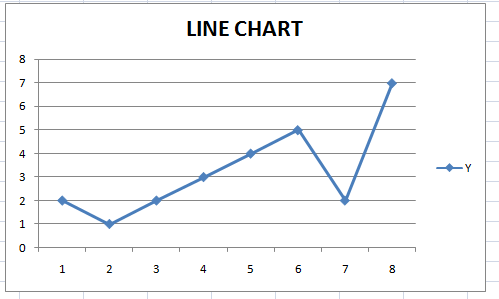# Line Chart Maker

Instructions: Use this line plot maker to create a line chart using the form below. All you have to do is type Y data (and optionally your X labels). Also, you can add a title a name to the axes.Y data (comma or space separated)X data (comma or space separated)Type the title (optional)Name of X variable (optional)Name of Y variable (optional)

## How to use this Line Chart Maker

More about the line charts: A line chart or line plot is a type of graphical representation with the characteristic that the data points are jointed by straight lines. This kind of setting makes sense in certain circumstances, like for instance in the case of a time series, but in order cases, a scatter plot could be more appropriate (in the case of majority of cross-sectional data).

### How do I create a line graph?

A line chart is constructed by joining a set of pairs $$(x_i,y_i)$$ by straight lines, starting from the points on the left, towards the right.

Graphically, a line chart looks like it follows:### Other graph makers

We provide other graph creators such as our normal probability grapher , our Pareto chart maker , time series plot maker or pie chart maker .

One specific type of line graph calculator is this ogive graph maker, which is essentially a line plot depicting a cumulative frequency table.